# Disjunctive normal form

(diff) ← Older revision | Latest revision (diff) | Newer revision → (diff)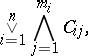(*)
where each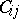(;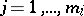) is either a variable or the negation of a variable. The form (*) is realizable if and only if, for each,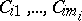do not contain both the formulasand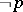, whereis any variable. For any propositional formulait is possible to construct an equivalent disjunctive normal formcontaining the same variables as. Such a formulais then said to be the disjunctive normal form of the formula.